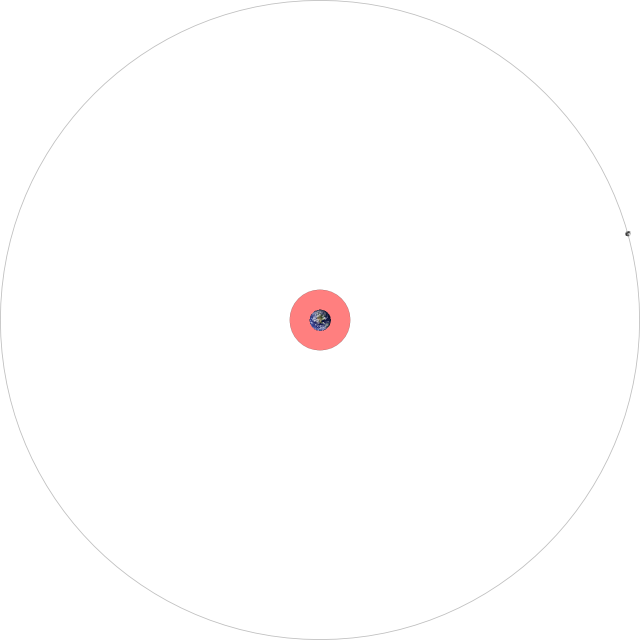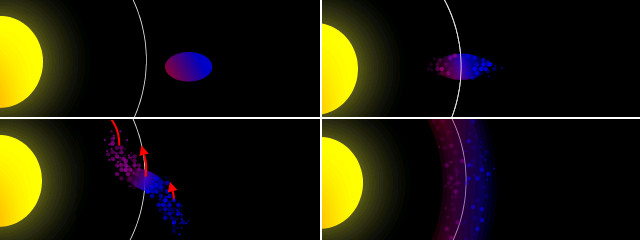# The Roche limit and planetary rings

As a satellite orbits around an object (a primary), the gravitational force on the side closest to the object is greater than that on the side opposite the object. This difference in gravitational attraction gives rise to a tidal force (so-called because it is what causes the tides on Earth). As a satellite approaches closer to the body it orbits this tidal force will eventually become greater than the gravitational forces holding the satellite together. The point at which this occurs is known as the Roche limit (named for Édouard Roche who first calculated it).

The Roche limit d depends on the radius of the primary RM, the density of the primary ρM and the density of the satellite ρm.$d = 2.44\; R_M \sqrt{\frac {\rho_M} {\rho_m}}$

If we take our Earth-Moon system as an example, with the radius of Earth being 6370 kilometres, the Earth’s density as 5520 kilograms per cubic metre and the Moon’s density as 3350 kg/m3 this gives us a Roche limit of 18 400 km. The Moon’s actual orbit is 385 000 km, so luckily we don’t have to worry about the Moon breaking up any time soon.The Earth-Moon system, to scale. The area beyond the Roche limit is shaded red.

When an orbiting object passes through the Roche limit it begins to break up, with the material closest to the object moving faster than the material behind it. This eventually leads to the formation of rings.